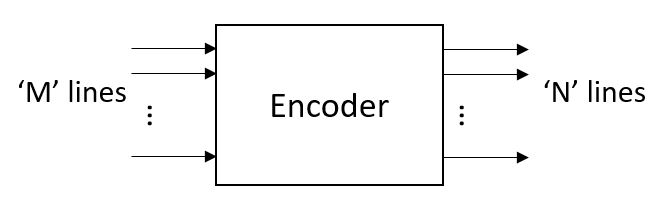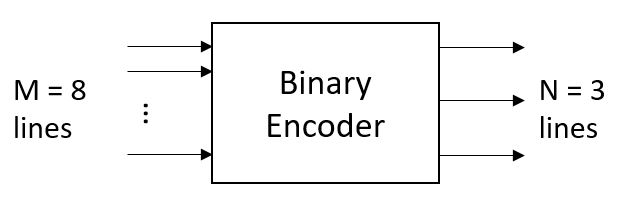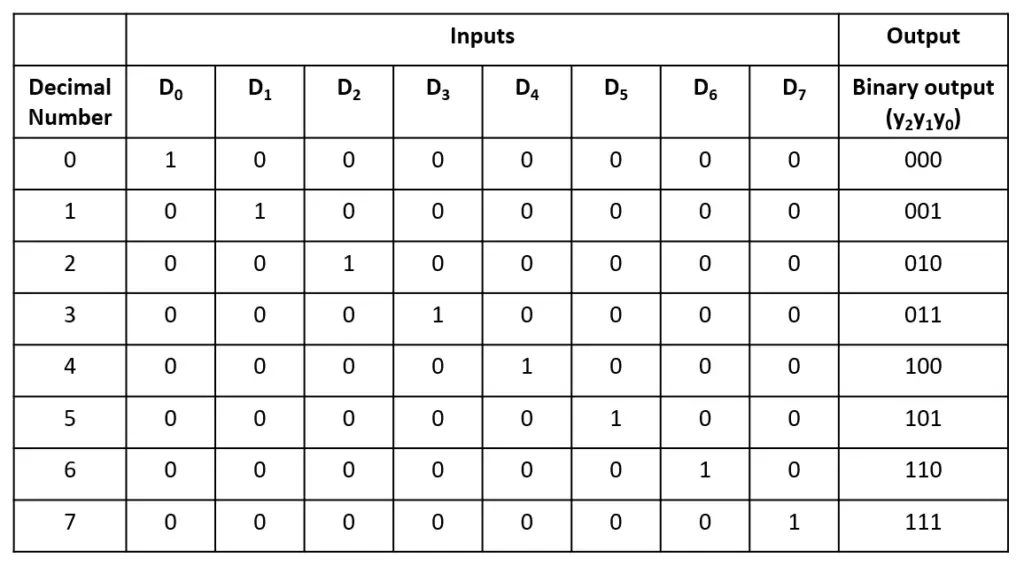Tutorials

An encoder basically converts ‘M’ input lines (can be decimal, hex, octal, etc) to coded ‘N’ output lines. Various encoders can be designed like decimal-to-binary encoders, octal-to-binary encoders, decimal- to-BCD encoders, etc

An encoder basically shrinks receiving many input data lines to the output lines. This is helpful in reducing input data lines for further processing.

## Block Diagram## Binary Encoder

The binary encoder converts M (=2^n) input lines to N (=n) coded binary code. It is also known as a digital encoder. A single input line must be high for valid coded output, otherwise, the output line will be invalid. To address this limitation, the priority encoder prioritizes each input line when multiple input lines are set to high.

Nomenclature: M: N encoder where M denotes input lines and N denotes coded output lines.

### 8:3 Binary Encoder

#### Block Diagram#### Truth Table#### Logical Expression

y2 = D4 + D5 + D6 + D7

y1 = D2 + D3 + D6 + D7

y0 = D1 + D3 + D5 + D7

#### 8:3 Binary Encoder Verilog Code

``````module binary_encoder(
input [7:0] D,
output [2:0] y);

assign y = D | D | D | D;
assign y = D | D | D | D;
assign y = D | D | D | D;
endmodule``````

#### Testbench Code

``````module tb;
reg [7:0] D;
wire [2:0] y;
int i;

binary_encoder bin_enc(D, y);

initial begin
D=8'b1; #1;
for(i=0; i<8; i++) begin
\$display("D = %h(in dec:%0d) -> y = %0h", D, i, y);
D=D<<1; #1;
end
end
endmodule``````

Output:

``````D = 01(in dec:0) -> y = 0
D = 02(in dec:1) -> y = 1
D = 04(in dec:2) -> y = 2
D = 08(in dec:3) -> y = 3
D = 10(in dec:4) -> y = 4
D = 20(in dec:5) -> y = 5
D = 40(in dec:6) -> y = 6
D = 80(in dec:7) -> y = 7``````

Verilog Codes